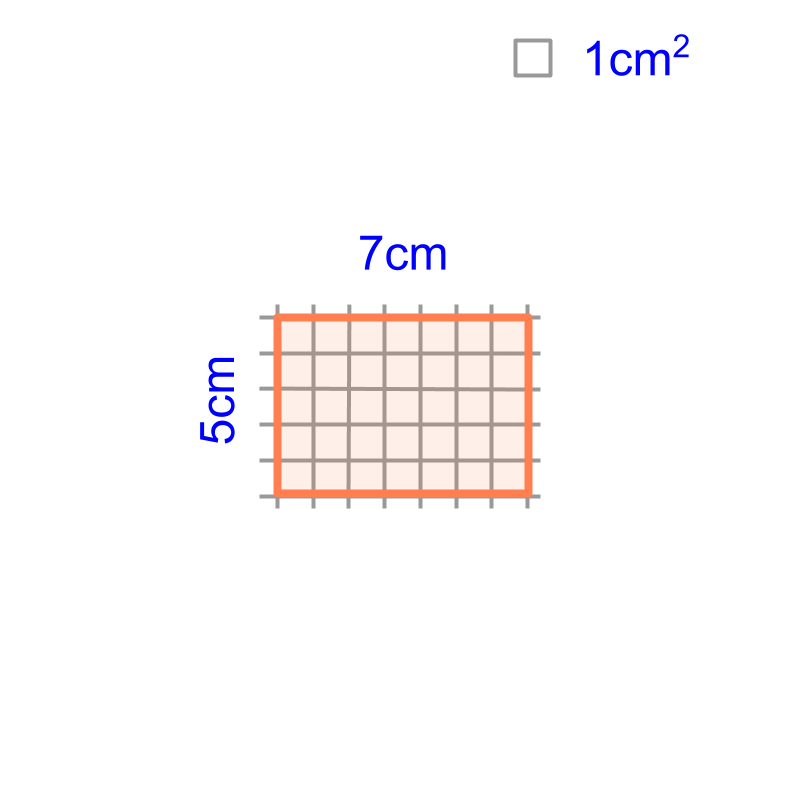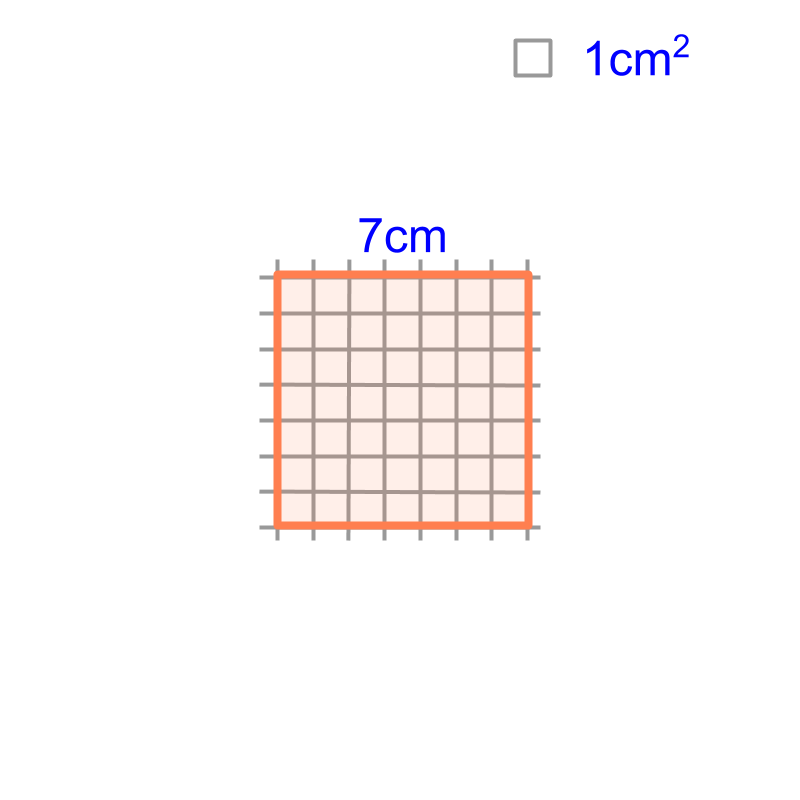maths > mensuration-basics

Area of Square and Rectangle

what you'll learn...

Overview

Area of a Square and a Rectangle :
Area of square $={\phantom{\rule{1ex}{0ex}}\text{side}}^{2}$$= {\textrm{s i \mathrm{de}}}^{2}$

Area of rectangle $=\phantom{\rule{1ex}{0ex}}\text{length}\phantom{\rule{1ex}{0ex}}×\phantom{\rule{1ex}{0ex}}\text{width}$$= \textrm{\le n > h} \times \textrm{w i \mathrm{dt} h}$Area of a figure is the "surface-span within a closed shape".The area of a square of side $7$$7$ cm is "$7×7=49c{m}^{2}$$7 \times 7 = 49 c {m}^{2}$". Area of a square is square of the side.The area of a rectangle of length $7$$7$ cm and width $5$$5$ cm is "$7×5=35c{m}^{2}$$7 \times 5 = 35 c {m}^{2}$".

Area of the rectangle is the product of its length and width.

What is the area of a rectangle with sides $2$$2$cm and $15$$15$mm?
The answer is "$2×1.5=3c{m}^{2}$$2 \times 1.5 = 3 c {m}^{2}$". The unit of measurement is made uniform before calculating the area.

summary

Area of a Square and a Rectangle :
Area of square $={\phantom{\rule{1ex}{0ex}}\text{side}}^{2}$$= {\textrm{s i \mathrm{de}}}^{2}$

Area of rectangle $=\phantom{\rule{1ex}{0ex}}\text{length}\phantom{\rule{1ex}{0ex}}×\phantom{\rule{1ex}{0ex}}\text{width}$$= \textrm{\le n > h} \times \textrm{w i \mathrm{dt} h}$Outline

The outline of material to learn "Mensuration basics : Length, Area, & Volume" is as follows.

•  Measuring Basics

→   Introduction to Standards

→   Measuring Length

→   Accurate & Approximate Meaures

→   Measuring Area

→   Measuring Volume

→   Conversion between Units of Measure

•  2D shapes

→   Perimeter of Polygons

→   Area of Square & rectangle

→   Area of Triangle

→   Area of Polygons

→   Perimeter and area of a Circle

→   Perimeter & Area of Quadrilaterals

•  3D shapes

→   Surface Area of Cube, Cuboid, Cylinder

→   Volume of Cube, Cuboid, Cylinder